International
Tables for
Crystallography
Volume C
Mathematical, physical and chemical tables
Edited by E. Prince

International Tables for Crystallography (2006). Vol. C, ch. 6.2, pp. 596-598

## Section 6.2.5. Special factors in the powder method

H. Lipson,a J. I. Langforda and H.-C. Hub

aSchool of Physics & Astronomy, University of Birmingham, Birmingham B15 2TT, England, and bChina Institute of Atomic Energy, PO Box 275 (18), Beijing 102413, People's Republic of China

### 6.2.5. Special factors in the powder method

| top | pdf |

In the powder method, all rays diffracted through an anglelie on the surface of a cone, and in the absence of preferred orientation the diffracted intensity is uniformly distributed over the circumference of the cone. The amount effective in blackening film, or intercepted by the receiving slit of a diffractometer, is thus inversely proportional to the circumference of the cone, and directly proportional to the fraction of the crystallites in a position to reflect. When allowance is made for these geometrical factors, it is found that for the Debye–Scherrer and diffractometer arrangements the intensity is proportional to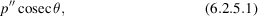where p′′ is the multiplicity factor (the number of permutations of hkl leading to the same value of). For the flat-plate front-reflection arrangement, the variation becomes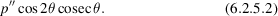Combining the polarization, angular-velocity, and special factors gives a trigonometric variation of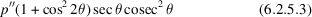for the Debye–Scherrer and diffractometer arrangements, and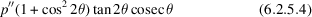for the flat-plate front-reflection arrangement.

Nowadays, angle-dispersive experiments are normally carried out by stepping the sample and detector in small angular increments, both being stationary while the intensity at each step is recorded. The Lorentz factor for a random powder sample is then of the form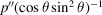. The factorarises from the fact that spherical shells of diffracted intensity in reciprocal space intersect the Ewald sphere at an angle that depends on, and the surface area of the shells increases as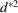, which is embodied in the factor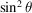. It turns out that this factor is equivalent to a combination of the polarization factor (6.2.2.1), the angular-velocity factor (6.2.3.1)and (6.2.5.1), and the form of (6.2.5.3)is thus unchanged.